﻿ 删边梯子图和“L”型梯子图的反强迫数

# 删边梯子图和“L”型梯子图的反强迫数The Anti-Forcing Numbers of the Edge Deleted Ladder Graphs and the “L” Type Ladder Graphs

Abstract: Through the classification calculation method, the anti-forcing number of the edge deleted ladder graphs is obtained, and the anti-forcing number of the “L” type ladder graphs is obtained. In the process, a combination proof of the Fibonacci sequence is obtained.

1. 引言

1997年，李学良研究了有强迫单边的六角系统  。2007年，Vukiěević和Trinajstić在处理有缺陷的苯类化合物的过程中推广了强迫单边的概念，提出了反强迫集和反强迫数的概念  。2016年雷洪川，叶永南和张和平引入了图的完美匹配的反强迫数的概念  。2008年Vukiěević和Trinajstić得出了卡塔型六角系统的反强迫数  。同年邓汉元给出了双链六角系统的反强迫数  。2011年张倩倩等人证明了卡塔型–亚苯基系统的反强迫数等于该系统中六角形的个数  。2013年蒋晓艳等人得到了硼氮富勒烯图的反强迫数  。2015年Hwang等引入了图的反强迫多项式的概念  。2017年邓凯和张和平证明了卡塔型六角系统的反强迫谱是连续的  。2018年姚海元等得到了循环梯状图完美匹配的反强迫谱和卢卡斯数列之间的关系  。2019年赵爽等加细了反强迫谱的概念，引入了反强迫多项式的概念，并计算了方格子等图类的反强迫多项式。总之，反强迫数的研究，大量的图类的反强迫谱/多项式有待计算  。本文主要研究了删边梯子图 $I{L}_{n}^{-i}$ 的反强迫数，得到了 $I{L}_{n}^{-i}$ 的反强迫谱。并通过收缩 $I{L}_{n}^{-i}$ 两条水平边的方法得到了“L”型梯子图的反强迫数，由此得到了几个与斐波那契数列和卢卡斯数列相关的结果。本文中将 $a\equiv b\left(\mathrm{mod}2\right)$ 简记为 $a\equiv b$，表示 $a,b$ 同奇偶。

2. 预备知识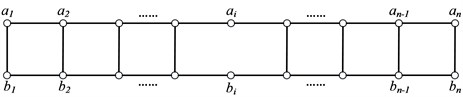Figure 1. The edge deleted ladder graphs $I{L}_{n}^{-i}$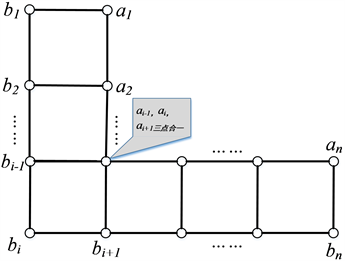Figure 2. “L” type ladder graphs $L{L}_{n}^{-i}$

$I{L}_{n}^{-i}$$L{L}_{n}^{-i}$ 中将形如 ${a}_{i}{a}_{i+1},{b}_{i}{b}_{i+1}$ 的边称为水平边，而形如 ${a}_{i}{b}_{i}$ 的边称为垂直边。当给定该图的一个完美匹配M，若 ${a}_{i}{a}_{i+1},{b}_{i}{b}_{i+1}\in M$，则将其称为水平匹配边；若 ${a}_{i}{b}_{i}\in M$，则将其称为垂直匹配边，垂直匹配边的总数记为p，特别的形如 ${a}_{j}{b}_{j}\in M\left(2\le j\le n-1\right)$ 称为内部垂直匹配边。

$af\left({L}_{n},M\right)=\left\{\begin{array}{ll}\frac{n}{2},\hfill & p=0\hfill \\ \frac{n+p-2}{2},\hfill & p\ge 1\hfill \end{array}$

$af\left(I{L}_{n}^{-i},M\right)=af\left({L}_{h},{M}_{1}\right)+af\left(I{L}_{n-h+1}^{-\left(i-h+1\right)},{M}_{2}\right)$

${a}_{h-1}{a}_{h},{a}_{h}{a}_{h+1},{b}_{h-1}{b}_{h},{b}_{h}{b}_{h+1}\notin M$。对于任意的M-交错圈 $C\in \mathcal{A}$${a}_{h-1}{a}_{h}$${a}_{h}{a}_{h+1}$ 不能同时在C中， ${b}_{h-1}{b}_{h}$${b}_{h}{b}_{h+1}$ 也不能同时在C中。若 ${a}_{h-1}{a}_{h}\in C$，则 ${a}_{h}{b}_{h},{b}_{h-1}{b}_{h}\in C$，此时 $C\in {\mathcal{A}}_{1}$ 是一个 ${M}_{1}$ -交错圈。同理，若 ${a}_{h}{a}_{h+1}\in C$，则 ${a}_{h}{b}_{h},{b}_{h}{b}_{h+1}\in C$，因此 $C\in {\mathcal{A}}_{2}$ 是一个 ${M}_{2}$ -交错圈。否则就有 ${a}_{h}{b}_{h}\notin C$。所以 $C\in \mathcal{A}$ 是一个 ${M}_{1}$ -交错圈或 ${M}_{2}$ -交错圈，因此 $\mathcal{A}\subseteq {\mathcal{A}}_{1}\cup {\mathcal{A}}_{2}$，即 $\mathcal{A}={\mathcal{A}}_{1}\cup {\mathcal{A}}_{2}$。对任意的 ${C}_{1},{C}_{2}\in \mathcal{A}$，若 ${C}_{1},{C}_{2}\in {\mathcal{A}}_{1}$ (或 ${\mathcal{A}}_{2}$ )，则 ${C}_{1},{C}_{2}$${M}_{1}$ -相容(或 ${M}_{2}$ -相容)的。不然${M}_{j}$ -相容的， $j=1,2$，故 ${c}^{\prime }\left(M\right)\le {c}^{\prime }\left({M}_{1}\right)+{c}^{\prime }\left({M}_{2}\right)$

${C}_{1},{C}_{2}\in {\mathcal{A}}_{j}$，则 ${C}_{1},{C}_{2}$ 是相容 ${M}_{j}$ -交错集， $j=1,2$，否则设 ${C}_{1}\in {\mathcal{A}}_{\text{1}}$${C}_{2}\in {\mathcal{A}}_{\text{2}}$。若 ${C}_{1}\cap {C}_{2}=\left\{{a}_{h}{b}_{h}\right\}$

${C}_{1}$${C}_{2}$ 是M-相容的。若 ${C}_{1},{C}_{2}$ 中最多有一个圈包含 ${a}_{h}{b}_{h}$，则 ${C}_{1}$${C}_{2}$ 不交， ${C}_{1}$${C}_{2}$ 也是M-相容的。因此，

${c}^{\prime }\left(M\right)\ge {c}^{\prime }\left({M}_{1}\right)+{c}^{\prime }\left({M}_{2}\right)$

$af\left(I{L}_{n}^{-i},M\right)=af\left({L}_{h},{M}_{1}\right)+af\left(I{L}_{n-h+1}^{-\left(i-h+1\right)},{M}_{2}\right)$

$Af\left(G,x\right)=\underset{M\in \mathcal{M}\left(G\right)}{\sum }{x}^{af\left(G,M\right)}$

$Af\left(G,x\right)\text{}=\underset{i=af\left(G\right)}{\overset{Af\left(G\right)}{\sum }}v\left(G,i\right){x}^{i}$

1) $Af\left(G,1\right)$ 表示G的完美匹配的个数；

2) ${\frac{\text{d}}{\text{d}x}Af\left(G,x\right)|}_{x=1}$ 表示G的所有完美匹配的反强迫数之和；

3) $\frac{{\frac{\text{d}}{\text{d}x}Af\left(G,x\right)|}_{x=1}}{Af\left(G,1\right)}$ 表示G的平均反强迫数。

3. $I{L}_{n}^{-i}$ 的反强迫数和反强迫谱

$af\left(I{L}_{n}^{-i},M\right)=\left\{\begin{array}{ll}\frac{n-2}{2},\hfill & \text{\hspace{0.17em}}\text{\hspace{0.17em}}\text{\hspace{0.17em}}\text{\hspace{0.17em}}\text{\hspace{0.17em}}\text{\hspace{0.17em}}p=0\hfill \\ \frac{n-1}{2},\hfill & p=1且i为偶数\hfill \\ \frac{n-3}{2},\hfill & p=1且i为奇数\hfill \\ \frac{n-2}{2},\hfill & \text{\hspace{0.17em}}\text{\hspace{0.17em}}\text{\hspace{0.17em}}\text{\hspace{0.17em}}\text{\hspace{0.17em}}\text{\hspace{0.17em}}p=2\hfill \end{array}$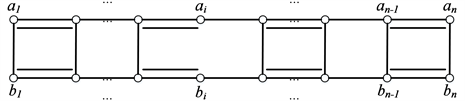Figure 3. The case $p=0$ in $I{L}_{n}^{-i}$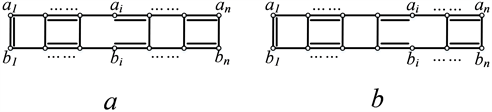Figure 4. The case $p=1$ (a) i is even; (b) i is odd

$\mathcal{A}$$I{L}_{n}^{-i}$ 的相容M-交错集，因为一个M-交错圈至少包含两条垂直边，当i是偶数时，所有交错4圈和交错圈 ${a}_{1}{a}_{2}\cdots {a}_{i}{a}_{i+1}{b}_{i+1}{b}_{i}\cdots {b}_{2}{b}_{1}{a}_{1}$ 是M-相容的，且用完了每一个垂直单边，又因包含 ${a}_{1}{b}_{1}$ 的M-相容交

$af\left(I{L}_{n}^{-i},M\right)=\left\{\begin{array}{ll}q=\frac{n-1}{2},\hfill & i为偶数\hfill \\ q-1=\frac{n-3}{2},\hfill & i为奇数\hfill \end{array}$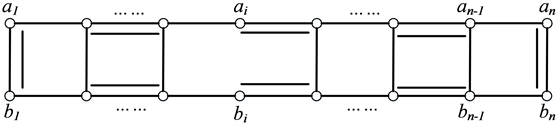Figure 5. $p=2$ in $I{L}_{n}^{-i}$

$af\left(I{L}_{n}^{-i},M\right)=q=\frac{n-2}{2}$

$I{L}_{n}^{-i}$ 中，我们把位于 ${a}_{i}{b}_{i}$ 左边的垂直匹配边的总数记为 ${p}_{1}$，位于 ${a}_{i}{b}_{i}$ 右边的垂直匹配边的总数记为 ${p}_{2}$，显然有 $p={p}_{1}+{p}_{2}\equiv n$

$af\left(I{L}_{n}^{-i},M\right)=\left\{\begin{array}{cc}\frac{n+p-2}{2},& {p}_{1}=0,且{a}_{i-1}{a}_{i}\in M或\text{ }{p}_{2}=0且{a}_{i}{a}_{i+1}\in M,\\ \frac{n+p-4}{2},& 其它.\end{array}$

$af\left(I{L}_{n}^{-i},M\right)=q-1=\frac{n-2}{2}=\frac{n+p-2}{2}$

$af\left(I{L}_{j}^{-i},{M}_{\text{1}}\right)=\left\{\begin{array}{ll}\frac{j-1}{2}，\hfill & i为偶数\hfill \\ \frac{j-3}{2}，\hfill & i为奇数\hfill \end{array}$

$af\left({L}_{n-j+1},{M}_{2}\right)=\frac{n-j+1+{p}_{2}-2}{2}$

$\begin{array}{c}af\left(I{L}_{n}^{-i},M\right)=af\left(I{L}_{j}^{-i},{M}_{\text{1}}\right)+af\left({L}_{n-j+1},{M}_{\text{2}}\right)\\ =\frac{j-1}{2}+\frac{n-j+1+{p}_{2}-2}{2}\\ =\frac{n+p-2}{2}\end{array}$

$\begin{array}{c}af\left(I{L}_{n}^{-i},M\right)=af\left(I{L}_{j}^{-i},{M}_{\text{1}}\right)+af\left({L}_{n-j+1},{M}_{\text{2}}\right)\\ =\frac{j-3}{2}+\frac{n-j+1+{p}_{2}-2}{2}\\ =\frac{n+p-4}{2}\end{array}$

${p}_{1}\ne 0,{p}_{2}=0$ 时同理可得

$af\left(I{L}_{n}^{-i},M\right)=\left\{\begin{array}{ll}\frac{n+p-2}{2},\hfill & {a}_{i}{a}_{i+1}为匹配边\hfill \\ \frac{n+p-4}{2},\hfill & {a}_{i-1}{a}_{i}为匹配边\hfill \end{array}$

$af\left({L}_{j},{M}_{\text{1}}\right)=\frac{j+{p}_{1}-2}{2}$

$af\left(I{L}_{k-j+1}^{-\left(i-j+1\right)},{M}_{\text{2}}\right)=\frac{k-j+1-2}{2}$

$af\left({L}_{n-k+1},{M}_{\text{3}}\right)=\frac{n-k+1+{p}_{2}-2}{2}$

$\begin{array}{c}af\left(I{L}_{n}^{-i},M\right)=af\left({L}_{j},{M}_{1}\right)+af\left(I{L}_{k-j+1}^{-\left(i-j+1\right)},{M}_{2}\right)+af\left({L}_{n-k+1},{M}_{3}\right)\\ =\frac{j+{p}_{1}-2}{2}+\frac{k-j+1-2}{2}+\frac{n-k+1+{p}_{2}-2}{2}\\ =\frac{n+p-4}{2}\end{array}$

$Spe{c}_{af}\left(I{L}_{n}^{-i}\right)=\left\{\begin{array}{ll}\left\{\frac{n-3}{2}+f,\frac{n-1}{2}+f,\cdots ,\frac{n+p-4}{2}+f,\cdots ,\frac{2n-5}{2}+f\right\},\hfill & n\ge 3且n为奇数\hfill \\ \left\{\frac{n-2}{2},\frac{n-2}{2}+f,\cdots ,\frac{n+p-4}{2}+f,\cdots ,\frac{2n-5}{2}+f\right\},\hfill & n\ge 4且n为偶数\hfill \end{array}$

$af\left(I{L}_{n}^{-i},M\right)=\frac{n-2}{2}$

$i\overline{)\equiv }j$ 时，

$af\left(I{L}_{n}^{-i},M\right)=\frac{n+p-2}{2}=\frac{n-1}{2}$

$i\equiv j$ 时，

$af\left(I{L}_{n}^{-i},M\right)=\frac{n+p-4}{2}=\frac{n-3}{2}$

$af\left(I{L}_{n}^{-i},M\right)=\frac{n+p-4}{2}+f=\frac{n-3}{2}+f$

$af\left(I{L}_{n}^{-i},M\right)=\frac{n+p-2}{2}=\frac{n+p-4}{2}+1=\frac{n+p-4}{2}+f$

$af\left(I{L}_{n}^{-i},M\right)=\frac{n+p-4}{2}=\frac{n+p-4}{2}+f$

$Af\left(I{L}_{n}^{-i},x\right)=\underset{p=1}{\overset{n-2}{\sum }}|{M}_{p}|{x}^{\frac{n+p-4}{2}}$

$Af\left(I{L}_{8}^{-3},x\right)=7{x}^{3}+9{x}^{4}+2{x}^{5}$

$Af\left(I{L}_{7}^{-4},x\right)=8{x}^{3}+4{x}^{4}$

$Af\left(I{L}_{16}^{-6},x\right)=19{x}^{7}+141{x}^{8}+303{x}^{9}+275{x}^{10}\text{+}120{x}^{11}+25{x}^{12}+2{x}^{13}$

$af\left(I{L}_{n}^{-i}\right)=\left\{\begin{array}{ll}\frac{n-3}{2}+f,\hfill & n\ge 3且n为奇数\hfill \\ \frac{n-2}{2},\hfill & n\ge 4且n为偶数\hfill \end{array}$

$|{M}_{p}|=\underset{{p}_{1}=0}{\overset{p}{\sum }}\left(\begin{array}{c}⌊\frac{i-1+{p}_{1}}{2}⌋\\ {p}_{1}\end{array}\right)\left(\begin{array}{c}⌊\frac{n-i+p-{p}_{1}}{2}⌋\\ p-{p}_{1}\end{array}\right)$

${a}_{i-1}{a}_{i}\in M$${p}_{1}$ 必为偶数，则 $I{L}_{n}^{-i}$ 可以从 ${a}_{i-2}{a}_{i-1}$${a}_{i}{a}_{i+1}$ 处分解开，由引理8知

${|M|}_{p}=\underset{\begin{array}{l}{p}_{1}=0\\ {p}_{1}\equiv i\end{array}}{\overset{p}{\sum }}\left(\begin{array}{c}\frac{i-2+{p}_{1}}{2}\\ {p}_{1}\end{array}\right)\left(\begin{array}{c}\frac{n-i+{p}_{2}}{2}\\ {p}_{2}\end{array}\right)$

${a}_{i-1}{a}_{i}\notin M$${p}_{1}$ 必为奇数，同理

${|M|}_{p}=\underset{\begin{array}{l}{p}_{1}=1\\ {p}_{1}\overline{)\equiv }i\end{array}}{\overset{p}{\sum }}\left(\begin{array}{c}\frac{i-2+{p}_{1}}{2}\\ {p}_{1}\end{array}\right)\left(\begin{array}{c}\frac{n-i+{p}_{2}}{2}\\ {p}_{2}\end{array}\right)$

${a}_{i-1}{a}_{i}\in M$${p}_{1}$ 必为奇数，

${|M|}_{p}=\underset{\begin{array}{l}{p}_{1}=1\\ {p}_{1}\equiv i\end{array}}{\overset{p}{\sum }}\left(\begin{array}{c}\frac{i-1+{p}_{1}}{2}\\ {p}_{1}\end{array}\right)\left(\begin{array}{c}\frac{n-i-1+{p}_{2}}{2}\\ {p}_{2}\end{array}\right)$

${a}_{i-1}{a}_{i}\notin M$${p}_{1}$ 必为偶数，

${|M|}_{p}=\underset{\begin{array}{l}{p}_{1}=0\\ {p}_{1}\overline{)\equiv }i\end{array}}{\overset{p}{\sum }}\left(\begin{array}{c}\frac{i-1+{p}_{1}}{2}\\ {p}_{1}\end{array}\right)\left(\begin{array}{c}\frac{n-i-1+{p}_{2}}{2}\\ {p}_{2}\end{array}\right)$

$\begin{array}{c}|{M}_{p}|=\underset{\begin{array}{l}{p}_{1}=0\\ {p}_{1}\equiv i\end{array}}{\overset{p}{\sum }}\left(\begin{array}{c}\frac{i-2+{p}_{1}}{2}\\ {p}_{1}\end{array}\right)\left(\begin{array}{c}\frac{n-i+p-{p}_{1}}{2}\\ p-{p}_{1}\end{array}\right)+\underset{\begin{array}{l}{p}_{1}=0\\ {p}_{1}\overline{)\equiv }i\end{array}}{\overset{p}{\sum }}\left(\begin{array}{c}\frac{i-1+{p}_{1}}{2}\\ {p}_{1}\end{array}\right)\left(\begin{array}{c}\frac{n-i-1+p-{p}_{1}}{2}\\ p-{p}_{1}\end{array}\right)\\ =\underset{{p}_{1}=0}{\overset{p}{\sum }}\left(\begin{array}{c}⌊\frac{i-1+{p}_{1}}{2}⌋\\ {p}_{1}\end{array}\right)\left(\begin{array}{c}⌊\frac{n-i+p-{p}_{1}}{2}⌋\\ p-{p}_{1}\end{array}\right)\end{array}$

$|{M}_{p}|=\left(\begin{array}{c}\frac{n+p}{2}\\ p\end{array}\right)-\underset{{p}_{1}=0}{\overset{z}{\sum }}\left(\begin{array}{c}\frac{i-1+{p}_{1}}{2}\\ {p}_{1}\end{array}\right)\left(\begin{array}{c}\frac{n-i+{p}_{2}}{2}\\ {p}_{2}\end{array}\right)$

$|M|={F}_{n+1}-{F}_{i}{F}_{n-i+1}=\underset{p=0}{\overset{n-2}{\sum }}|{M}_{p}|$

4. “L”型梯子图 $L{L}_{n}^{-i}$ 的反强迫数

$L{L}_{n}^{-i}$ 是收缩 $I{L}_{n}^{-i}$${a}_{i-1}{a}_{i}$${a}_{i}{a}_{i+1}$ 两条边得到的“L”型梯子图。任取 $I{L}_{n}^{-i}$ 的完美匹配 ${M}^{i}$，必有 ${a}_{i-1}{a}_{i},{a}_{i}{a}_{i+1}$ 之一(记为e)属于 ${M}^{i}$，则 $M={M}^{i}-e$$L{L}_{n}^{-i}$ 的完美匹配，反之亦然。因而 $I{L}_{n}^{-i}$ 中的 ${M}^{i}$ -交错圈和 $L{L}_{n}^{-i}$ 中的M-交错圈一一对应。进一步我们有

$af\left(I{L}_{n}^{-i},{M}^{i}\right)\ge af\left(L{L}_{n}^{-i},M\right)$

$af\left(I{L}_{n}^{-i},{M}^{i}\right)\le af\left(L{L}_{n}^{-i},M\right)$

$af\left(L{L}_{n}^{-i},M\right)=\left\{\begin{array}{cc}\frac{n+p-2}{2}，& {p}_{1}=0且{b}_{i-1}{b}_{i}\in M或{p}_{2}=0且{b}_{i}{b}_{i+1}\in M,\\ \frac{n+p-4}{2}，& 其它.\end{array}$

NOTES

*通讯作者。

 Li, X. (1997) Hexagonal Systems with Forcing Single Edges. Discrete Applied Mathematics, 72, 295-301.
https://doi.org/10.1016/0166-218X(95)00116-9

 Vukiěević, D. and Trinajstić, N. (2007) On the Anti-Forcing Number of Benzenoids. Journal of Mathematical Chemistry, 42, 575-583.
https://doi.org/10.1007/s10910-006-9133-6

 Lei, H., Yeh, Y. and Zhang, H. (2016) Anti-Forcing Numbers of Perfect Matchings of Graphs. Discrete Applied Mathematics, 202, 95-105.
https://doi.org/10.1016/j.dam.2015.08.024

 Vukiěević, D. and Trinajstić, N. (2008) On the Anti-Kekule Number and Anti-Forcing Number of Cata-Condensed Benzenoids. Mathematics in Chemistry, 43, 719-726.
https://doi.org/10.1007/s10910-006-9223-5

 Deng, H. (2008) The Anti-Forcing Number of Double Hexagonal Chains. MATCH—Communications in Mathematical and in Computer Chemistry, 60, 183-192.

 Zhang, Q., Bian, H. and Vumar, E. (2011) On the Anti-Kekule Number and Anti-Forcing Number of Cata Condensed Phenylenes. MATCH—Communications in Mathematical and in Computer Chemistry, 65, 799-806.

 蒋晓艳, 程晓胜. 硼氮富勒烯图的反强迫数[J]. 湖南师范学院学报: 自然科学版, 2013, 33(3): 28-30.

 Hwang, H.-K., Lei, H., Yeh, Y.-N. and Zhang, H. (2015) Distribution of Forcing and Anti-Forcing Numbers of Random Perfect Matchings on Hexagonal Chains and Crowns. http://140.109.74.92/hk/?p=873

 Deng, K. and Zhang, H. (2017) Anti-Forcing Spectrum of Any Cata-Condensed Hexagonal System Is Continuous. Frontiers of Mathematics in China, 12, 325-337.
https://doi.org/10.1007/s11464-016-0605-0

 姚海元, 王杰彬, 王旭. 循环梯状图的完美匹配的反强迫谱与卢卡斯数[J]. 西北师范大学学报: 自然科学版, 2018, 54(2): 21-25.

 Zhao, S. and Zhang, H. (2019) Forcing and Anti-Forcing Polynomials of Perfect Matchings for Some Rectangle Grids. Journal of Mathematical Chemistry, 57, 202-225.
https://doi.org/10.1007/s10910-018-0944-z

Top